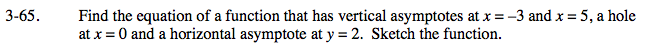### Home > CALC > Chapter 3 > Lesson 3.2.2 > Problem3-65

3-65.

Find the equation of a function that has vertical asymptotes at x = −3 and x = 5, a hole at x = 0 and a horizontal asymptote at y = 2. Sketch the function. Homework Help ✎To guarantee the locations of holes and vertical asymptotes, write a rational function in factored form:

The function above has a horizontal asymptote of y = 0. This is because the highest power in the nurerator is smaller than the highest power in the denominator. How can you transform the function so that the horizontal asymptote vertically shifts to y = 2?